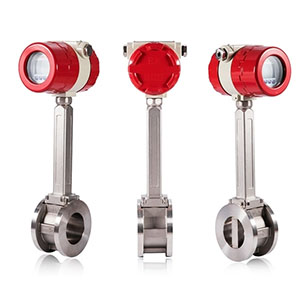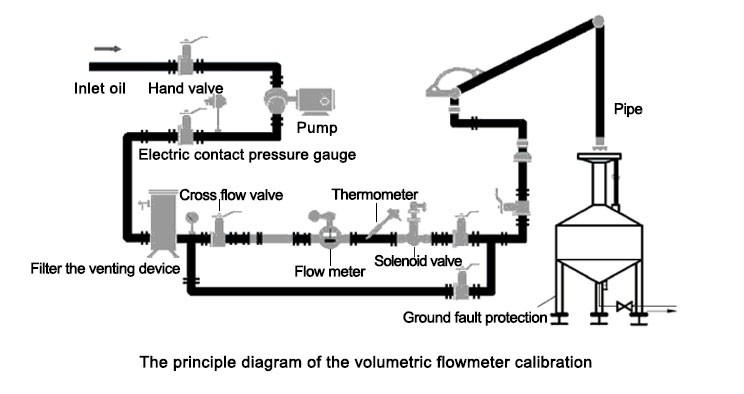# Flow Meter Calibration

Flow is the amount of fluid passing through the cross-section of a pipe or device per unit time. This quantity is expressed in mass, called mass flow; expressed in volume, expressed in weight called volume flow, called gravimetric flow. Since the density of the fluid varies with the state parameters of the fluid, the state of the fluid must be given while the volume flow is given. Especially for gases, since the density changes significantly with pressure and temperature, in order to easily compare the volume flow, the volume flow in the working state is mostly converted into the volume flow in the standard state (temperature 20°C, absolute pressure 101325Pa). Commonly used flow measurement methods include velocity flow measurement methods, volumetric flow measurement methods, and direct or indirect methods to measure the fluid mass flowing through the pipe section in unit time. A number of velocity-type flow measurement methods are used in the above-mentioned methods. Except that the applicable flow meter does not need to be verified by the standard throttling device, almost all the other flow meters must be verified before leaving the factory. Always calibrate while the flow meter is in use.

Except for the standard throttling device that does not need to be tested experimentally, the flow meters used are almost always tested when they leave the factory. Calibration should also be performed frequently during the use of the flow meter. The calibration methods of liquid flow meters mainly include volume method, mass method, standard volume tube method and standard flow meter comparison method.## Volumetric method

The volumetric method is the most commonly used. Volumetric flow measurement is the use of a fixed small volume to repeatedly measure the volume of fluid passing through the flow meter. Therefore, there must be a space that constitutes a standard volume inside the positive displacement flow meter, which is usually called the "measurement space" or "measurement chamber" of the positive displacement flow meter. This space is formed by the inner wall of the meter housing and the flow meter rotating parts. The working principle of the positive displacement flow meter is: when the fluid passes through the flow meter, a certain pressure difference will be generated between the inlet and outlet of the flow meter. The rotating part of the flow meter (referred to as the rotor) rotates under the action of this pressure difference and discharges the fluid from the inlet to the outlet. During this process, the fluid fills the "metering space" of the flow meter again and again, and is then continuously sent to the outlet. Under the conditions of a given flow meter, the volume of the metering space is determined as long as the number of rotations of the rotor is measured. The cumulative value of the volume of fluid passing through the flow meter can then be obtained.

## Quality method

The mass method is a method of weighing the mass of the fluid flowing into the container during the measurement time to obtain the flow rate. During calibration, pump out the test fluid from the liquid storage container through the calibrated flow meter and enter the container containing the liquid, measure the temperature of the fluid while weighing the weight, and use it to determine the density value of the measured fluid at this temperature. The volume of the fluid can be obtained by dividing the weight of the fluid to be measured by the density of the fluid at the measured temperature. By comparing it with the volume indication (accumulated pulse number) of the meter, the meter constant and accuracy of the flow meter to be calibrated can be determined. The accuracy of this method can reach 0.1%.## Standard volume tube method

The working principle of the standard volume tube method is based on the volume method (standard volume method), but it is a dynamic measurement.

## Standard Flow meter Comparison Method

The standard flow meter comparison method is to connect the calibrated flow meter and the standard flow meter in series on the pipeline flowing through the test fluid, and obtain the error by comparing the measured values of the two. The accuracy of the standard flow meter is 2~3 times higher than that of the calibrated flow meter

## What are the calibration procedures for flow calibrators?

Main instrument calibration procedure

Master meter calibration is the process of comparing the measurements of the flow meter under test with the measurements of a calibrated flow meter or a "master" flow meter operating to the desired flow standard, and adjusting the calibration accordingly. Primary flow meters are usually devices calibrated to international standards. To perform a master meter calibration:
• Connect the main meter and the flow meter under test in series.
• Using the measured liquid volume, compare the readings of the main flow meter and the flow meter.
• Find the flow rate of the aliquoted sample by dividing its volumetric weight by the test duration.

Weight calibration procedure

Gravimetric calibration is one of the accurate and economical procedures for calibrating volumetric and mass flow meters. The gravimetric method is very suitable for the calibration of liquid flow calibrators in the petroleum, water purification, and petrochemical industries. To perform a gravity calibration, do the following:
• Place a sample (small portion) of process fluid in the tester and flow for 60 seconds while measuring the exact time.
• Use a calibrated scale to accurately measure the weight of the test fluid.
• After the test period is over, transfer the test fluid to the drain container.
• Find the flow rate of the aliquoted sample by dividing its volumetric weight by the test duration.
Compare the calculated flow to the flow meter's flow and adjust to the measured flow.

Piston calibration procedure

When a flow meter is used for mixed-phase fluid calibration to calibrate the total flow of solid-liquid mixed-phase fluids (such as water containing fine sand), if a flow meter calibrated by a single-phase electro-liquid phase is used, it will cause a calibration difference. Install the sensor at the pipe section that is likely to cause solid-liquid phase separation.